# Quickstart¶We are glad this package caught your attention, so let’s try to briefly showcase its power.

## Data¶

In this tutorial, we will use a historical Rossmann sales dataset that we load via the hcrystalball.utils.get_sales_data function.

A description of the dataset and available columns is given in the docstring.

:

import pandas as pd
import matplotlib.pyplot as plt
plt.style.use('seaborn')
plt.rcParams['figure.figsize'] = [12, 6]

:

from hcrystalball.utils import get_sales_data

df = get_sales_data(n_dates=100,
n_assortments=2,
n_states=2,
n_stores=2)

:

df

:

Store Sales Open Promo SchoolHoliday StoreType Assortment Promo2 State HolidayCode
Date
2015-04-23 817 17520 True False False a a False BE DE-BE
2015-04-23 251 16573 True False False a c False NW DE-NW
2015-04-23 335 11189 True False False b a True NW DE-NW
2015-04-23 380 10761 True False False a a True NW DE-NW
2015-04-23 788 15793 True False False a c False BE DE-BE
... ... ... ... ... ... ... ... ... ... ...
2015-07-31 523 15349 True True True c c False BE DE-BE
2015-07-31 513 19959 True True True a a False BE DE-BE
2015-07-31 380 17133 True True True a a True NW DE-NW
2015-07-31 335 17867 True True True b a True NW DE-NW
2015-07-31 251 22205 True True True a c False NW DE-NW

800 rows × 10 columns

## Define search space¶

Next step is to define ModelSelector for which frequency the data will be resampled to, how many steps ahead the forecast should run, and optionally define column, which contains ISO code of country/region to take holiday information for given days

Once done, creating grid search with possible exogenous columns and extending it with custom models

:

from hcrystalball.model_selection import ModelSelector

ms = ModelSelector(horizon=10,
frequency='D',
country_code_column='HolidayCode',
)

ms.create_gridsearch(sklearn_models=True,
n_splits = 2,
between_split_lag=None,
sklearn_models_optimize_for_horizon=False,
autosarimax_models=False,
prophet_models=False,
tbats_models=False,
exp_smooth_models=False,
average_ensembles=False,
stacking_ensembles=False,
exog_cols=['Open','Promo','SchoolHoliday','Promo2'],
#                     holidays_days_before=2,
#                     holidays_days_after=1,
#                     holidays_bridge_days=True,
)

:

from hcrystalball.wrappers import get_sklearn_wrapper
from sklearn.linear_model import LinearRegression



## Run model selection¶

By default the run will partition data by partition_columns and do for loop over all partitions.

If you have a problem, that make parallelization overhead worth trying, you can also use parallel_columns - subset of partition_columns over which the parallel run (using prefect) will be started.

If expecting the run to take long, it might be good to directly store results. Here output_path and persist_ methods might come convenient

:

# from prefect.engine.executors import LocalDaskExecutor
ms.select_model(df=df,
target_col_name='Sales',
partition_columns=['Assortment', 'State','Store'],
#                 parallel_over_columns=['Assortment'],
#                 persist_model_selector_results=False,
#                 output_path='my_results',
)


## Look at the results¶

Naturaly we are interested in which models were chosen, so that we can strip our parameter grid from the ones, which were failing and extend with more sophisticated models from most selected classes

:

ms.plot_best_wrapper_classes();

:

<AxesSubplot:title={'center':'Most often selected classes'}>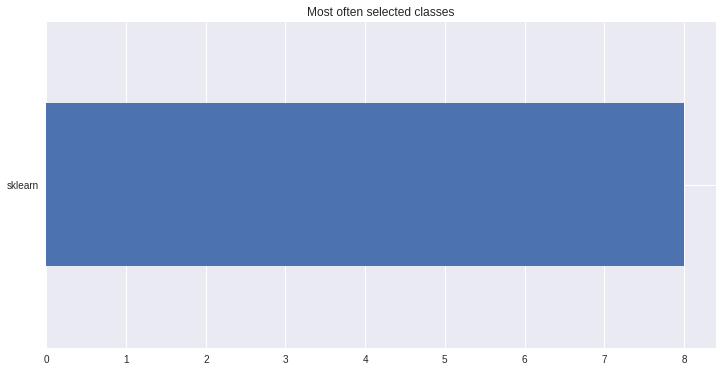There also exists convenient method to plot the results over all (or subset of) the data partitions to see how well our model fitted the data during cross validation

:

ms.plot_results(plot_from='2015-06-01');

:

[<AxesSubplot:title={'center':'Assortment=a State=NW Store=335'}>,
<AxesSubplot:title={'center':'Assortment=a State=NW Store=380'}>,
<AxesSubplot:title={'center':'Assortment=a State=BE Store=513'}>,
<AxesSubplot:title={'center':'Assortment=a State=BE Store=817'}>,
<AxesSubplot:title={'center':'Assortment=c State=NW Store=251'}>,
<AxesSubplot:title={'center':'Assortment=c State=BE Store=523'}>,
<AxesSubplot:title={'center':'Assortment=c State=NW Store=756'}>,
<AxesSubplot:title={'center':'Assortment=c State=BE Store=788'}>]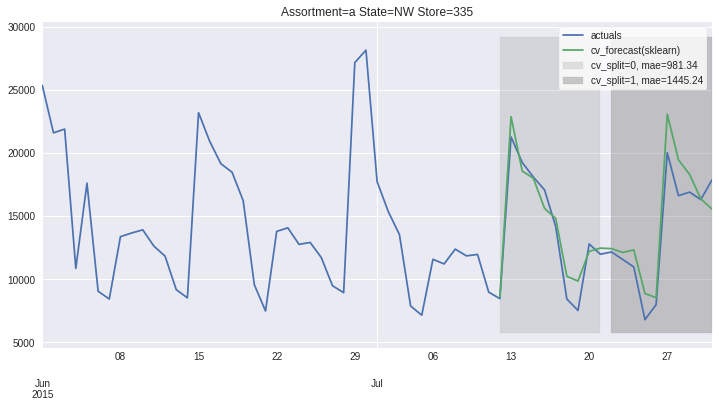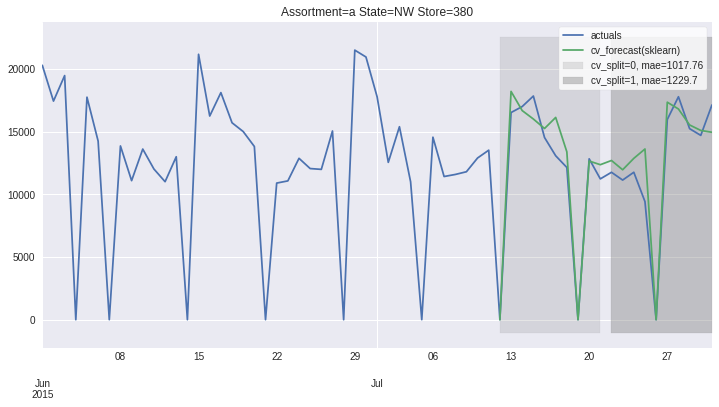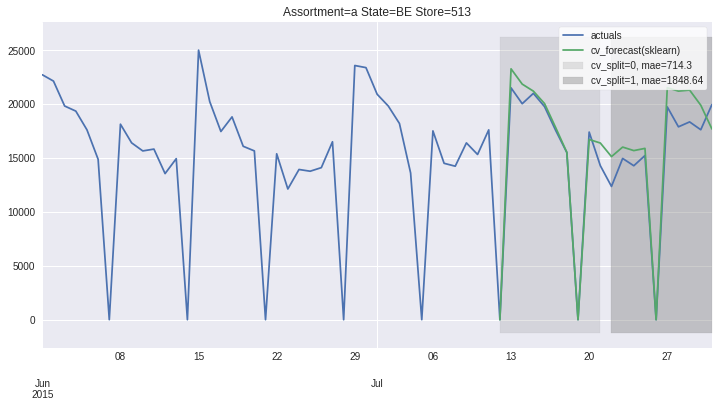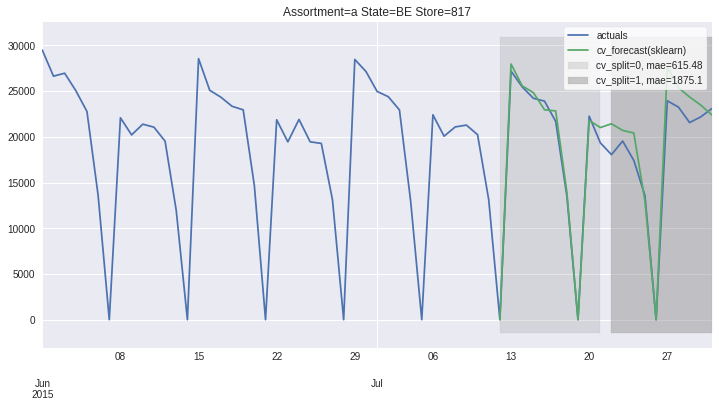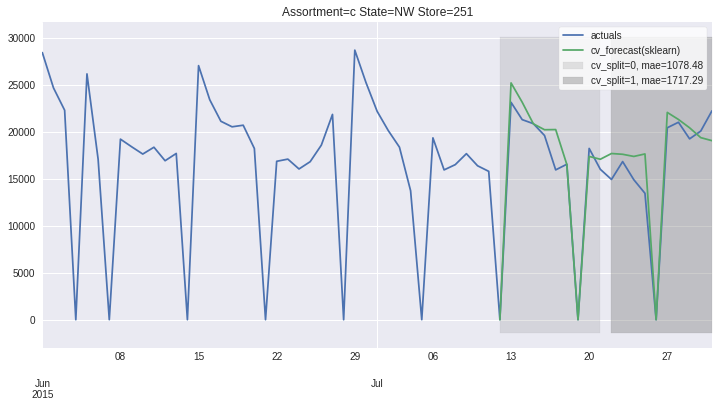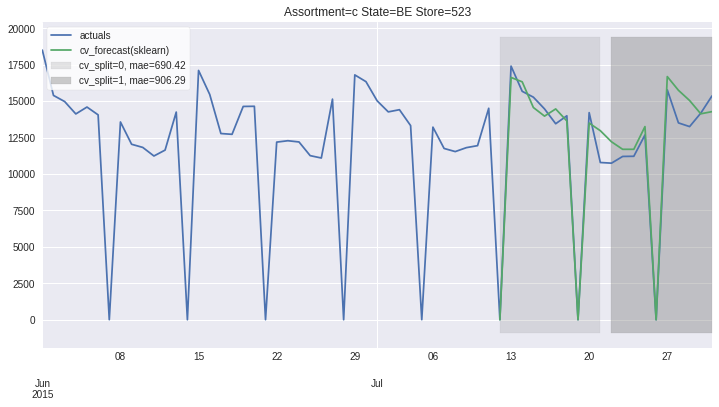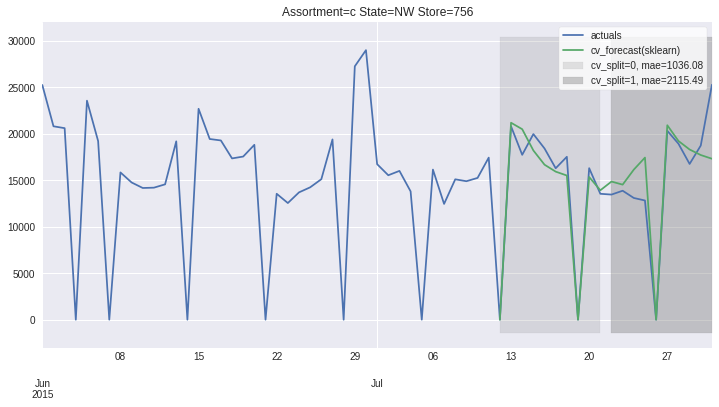## Accessing 1 Time-Series¶

To get to more information, it is advisable to go from all partitions level (ModelSelector) to single partition level (ModelSelectorResult).

ModelSelector stores results as a list of ModelSelectorResult objects in self.results. Here we provide rich __repr__ that hints on what information are available.

Another way to get the ModelSelectorResult is to use ModelSelector.get_result_for_partition that ensures the same results also when loading the stored results. Here the list access method fails (ModelSelector.results), because each ModelSelectorResults is stored with partition_hash name and later load ingests these files in alphabetical order.

Accessing training data to see what is behind the model, cv_results to check the fitting time or how big margin my best model had over the second best one or access model definition and explore its parameters are all handy things that we found useful

:

ms.results

:

ModelSelectorResult
-------------------
best_model_name: sklearn
frequency: D
horizon: 10

country_code_column: HolidayCode

partition: {'Assortment': 'a', 'State': 'NW', 'Store': 335}
partition_hash: 0d8965bc23395b49a81bdc3834bc15fb

df_plot: DataFrame (100, 6) suited for plotting cv results with .plot()
X_train: DataFrame (100, 6) with training feature values
y_train: DataFrame (100,) with training target values
cv_results: DataFrame (19, 16) with gridsearch cv info
best_model_cv_results: Series with gridsearch cv info
cv_data: DataFrame (20, 21) with models predictions, split and true target values
best_model_cv_data: DataFrame (20, 3) with model predictions, split and true target values

model_reprs: Dict of model_hash and model_reprs
best_model_hash: 49d81b3d670ac10c4084a1f390c82b3e
best_model: Pipeline(memory=None,
steps=[('exog_passthrough',
TSColumnTransformer(n_jobs=None, remainder='drop',
sparse_threshold=0.3,
transformer_weights=None,
transformers=[('raw_cols', 'passthrough',
['Open', 'Promo',
'SchoolHoliday', 'Promo2',
'HolidayCode'])],
verbose=False)),
('holiday',
Pipeline(memory=None,
steps=[('holiday_HolidayCode',
HolidayTransformer(bridge_days=...
max_depth=6,
max_features='auto',
max_leaf_nodes=None,
max_samples=None,
min_impurity_decrease=0.0,
min_impurity_split=None,
min_samples_leaf=1,
min_samples_split=2,
min_weight_fraction_leaf=0.0,
n_estimators=100, n_jobs=None,
name='sklearn',
oob_score=False,
optimize_for_horizon=False,
random_state=42, verbose=0,
warm_start=False))],
verbose=False))],
verbose=False)
-------------------

:

res = ms.get_result_for_partition(partition=ms.results.partition)


### Plotting results and errors for 1 time series¶

On this level we can also access the forecast plots - one that we already know with cv_forecasts and one that gives us only errors

:

res.plot_result(plot_from = '2015-06-01', title='forecasts');

:

<AxesSubplot:title={'center':'forecasts'}>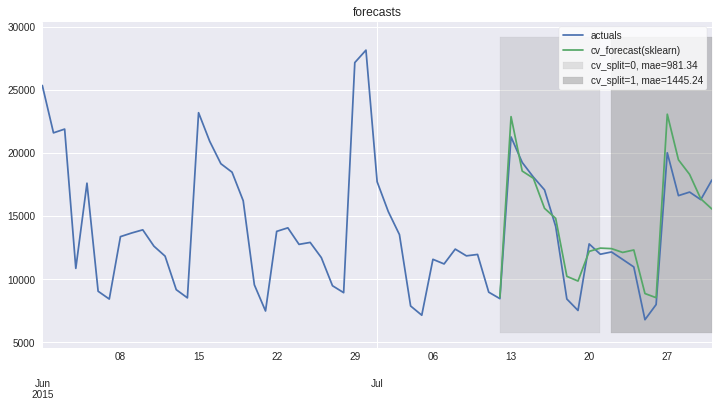:

res.plot_error(title='Errors');

:

cv_split_str
cv_split=0, mae=981.34     AxesSubplot(0.125,0.125;0.775x0.755)
cv_split=1, mae=1445.24    AxesSubplot(0.125,0.125;0.775x0.755)
Name: error, dtype: object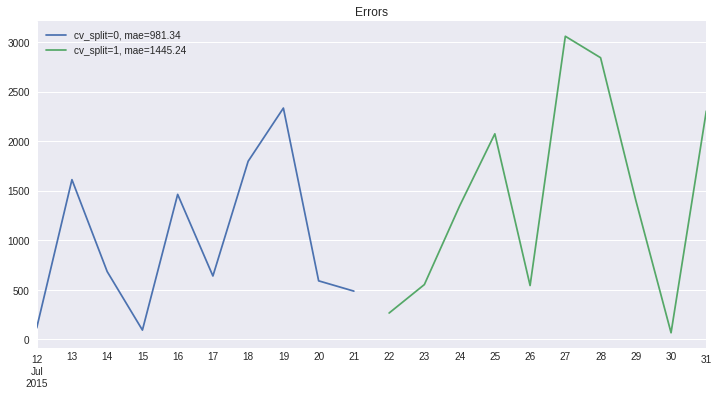To enable later usage of our found results, there are plenty of methods that can help storing and loading the results of model selection in a uniform way.

Some methods and functions persits/load the whole objects (load_model_selector, load_model_selector_result), while some provide direct access to the part we might only care if we run in production and have space limitations (load_best_model, load_partition, …)

:

from hcrystalball.model_selection import load_model_selector

res.persist(path='tmp')

ms.persist_results(folder_path='tmp/results')

:

ms = load_model_selector(folder_path='tmp/results')

:

ms.plot_results(plot_from='2015-06-01');

:

[<AxesSubplot:title={'center':'Assortment=a State=NW Store=380'}>,
<AxesSubplot:title={'center':'Assortment=c State=BE Store=523'}>,
<AxesSubplot:title={'center':'Assortment=c State=NW Store=251'}>,
<AxesSubplot:title={'center':'Assortment=a State=BE Store=513'}>,
<AxesSubplot:title={'center':'Assortment=c State=NW Store=756'}>,
<AxesSubplot:title={'center':'Assortment=c State=BE Store=788'}>,
<AxesSubplot:title={'center':'Assortment=a State=NW Store=335'}>,
<AxesSubplot:title={'center':'Assortment=a State=BE Store=817'}>]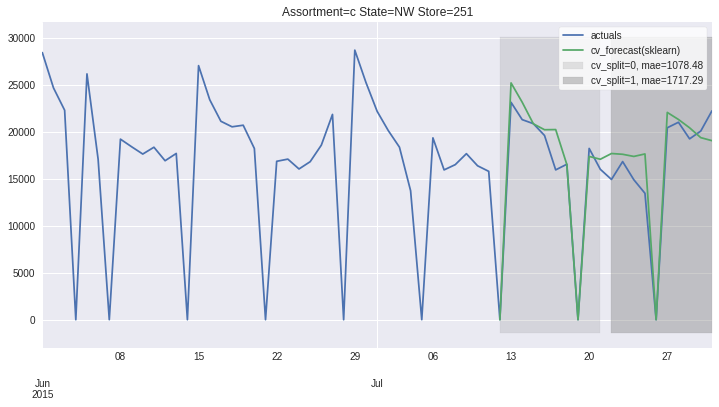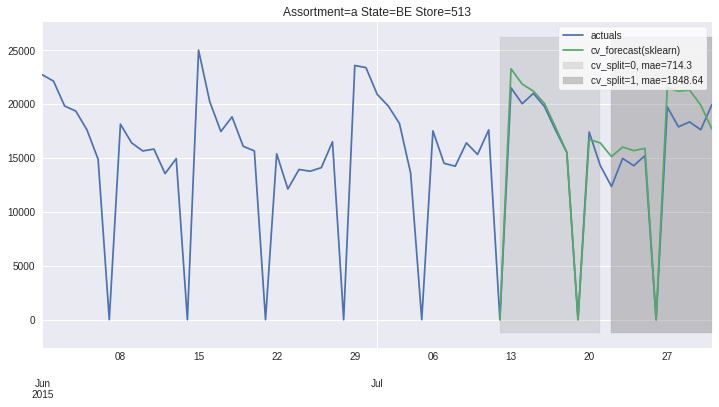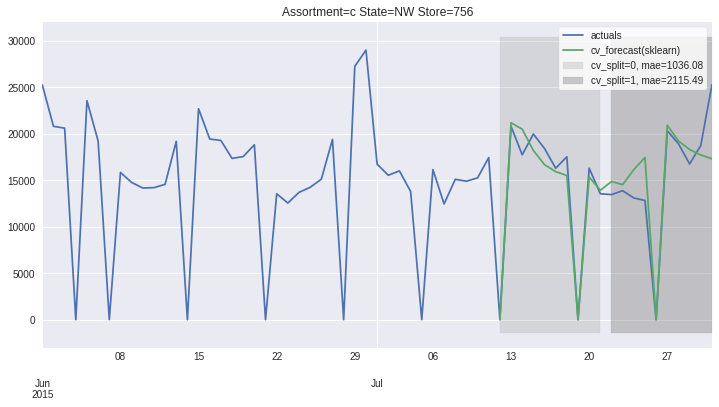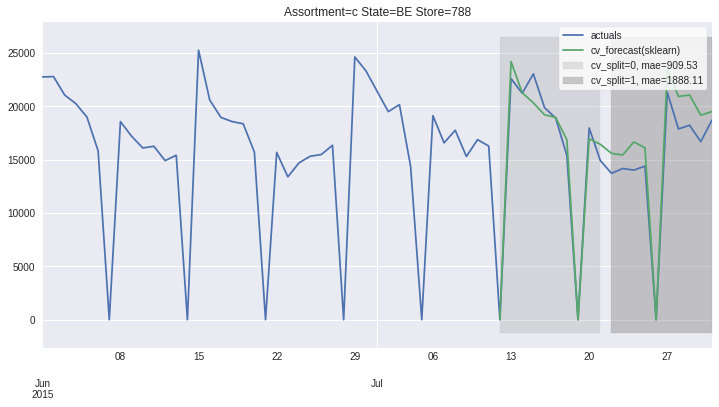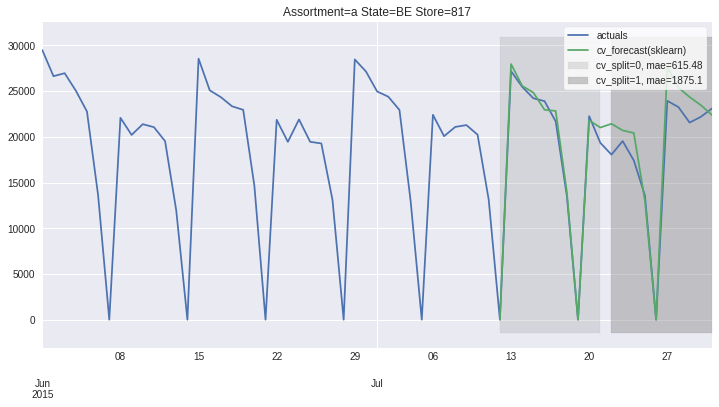:

# cleanup
import shutil
try:
shutil.rmtree('tmp')
except:
pass